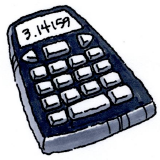### Home > CC2 > Chapter Ch1 > Lesson 1.1.5 > Problem1-45

1-45.

Copy the rows of equations below and write what you predict will be the next five rows in the sequence.

$1·9+2=11$
$12·9+3=111$
$123·9+4=1111$
$1234·9+5=11111$

1. What patterns do you see? Write your answer in complete sentences.

Look at the equations in separate parts, then compare each row.

In complete sentences, write about the patterns that you see from one row to the next.

The added number increases by $1$. The first factor in the multiplication adds another digit, the next consecutive number. The answers add a digit of one as they increase.

$12345·9+6=111111$
$123456·9+7=1111111$
$1234567·9+8=11111111$
$12345678·9+9=111111111$
$123456789·9+10=1111111111$

2.Use a calculator to discover whether your predictions were correct. If they were not correct, look at the pattern again and figure out how it is changing.